How Cheenta works to ensure student success?
Explore the Back-Story

# Eigen Value of a matrix | IIT JAM 2017 | Problem 58Try this problem from IIT JAM 2017 exam (Problem 58) and know how to evaluate Eigen value of a Matrix.

## Eigen value of a Matrix | IIT JAM 2017 | Problem 58

Let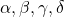be the eigenvalues of the matrix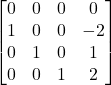Then find the value of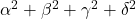### Key Concepts

Linear Algebra

Matrix

Eigen Values

Answer: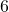IIT JAM 2017 , Problem 58

## Try with Hints

Characteristic Equation of a matrixof order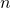is defined by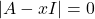, where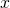is scalar and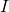is the identity matrix of order.

The roots of the characteristic equation are called the Eigen Values of that matrix.

Now it is easy. Give it a try.

Let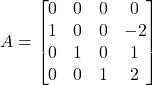Then its characteristic equation isfor some scalarandthe identity matrix of orderi.e.,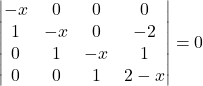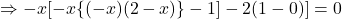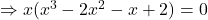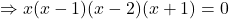Then the eigen values are :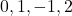Then,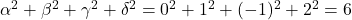[ANS]

## Subscribe to Cheenta at Youtube

Try this problem from IIT JAM 2017 exam (Problem 58) and know how to evaluate Eigen value of a Matrix.

## Eigen value of a Matrix | IIT JAM 2017 | Problem 58

Letbe the eigenvalues of the matrixThen find the value of### Key Concepts

Linear Algebra

Matrix

Eigen Values

Answer:IIT JAM 2017 , Problem 58

## Try with Hints

Characteristic Equation of a matrixof orderis defined by, whereis scalar andis the identity matrix of order.

The roots of the characteristic equation are called the Eigen Values of that matrix.

Now it is easy. Give it a try.

LetThen its characteristic equation isfor some scalarandthe identity matrix of orderi.e.,Then the eigen values are :Then,[ANS]

## Subscribe to Cheenta at Youtube

This site uses Akismet to reduce spam. Learn how your comment data is processed.

### Knowledge Partner